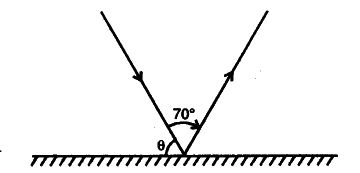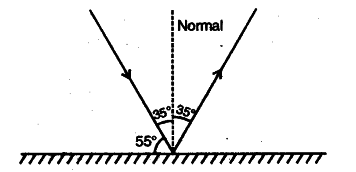# The angle between an incident ray and the mirror is as shown below

The angle between an incident ray and the mirror is as shown below. The total angle turned by the ray of light is 70^{ 0 }.What is the value of \theta ?The ray diagram is redrawn as shown below :Since the angle of incidence = angle of reflection,
therefore, \theta = 90° - 35° = 55^{ 0 }.